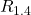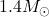N3AS-22-024

# Prospects for a precise equation of state measurement from Advanced LIGO and Cosmic Explorer

Daniel Finstad, Laurel V. White, Duncan A. Brown.
.

View

Abstract

Gravitational-wave observations of neutron star mergers can probe the nuclear equation of state by measuring the imprint of the neutron star’s tidal deformability on the signal. We investigate the ability of future gravitational-wave observations to produce a precise measurement of the equation of state from binary neutron star inspirals. Since measurability of the tidal effect depends on the equation of state, we explore several equations of state that span current observational constraints. We generate a population of binary neutron stars as seen by a simulated Advanced LIGO-Virgo network, as well as by a planned Cosmic Explorer observatory. We perform Bayesian inference to measure the parameters of each signal, and we combine measurements across each population to determine, the radius of aneutron star. We find that with 321 signals the LIGO-Virgo network is able to measureto better than 2% precision for all equations of state we consider, however we find that achieving this precision could take decades of observation, depending on the equation of state and the merger rate. On the other hand we find that with one year of observation, Cosmic Explorer will measureto better than 0.6% precision. In both cases we find that systematic biases, such as from an incorrect mass prior, can significantly impact measurement accuracy and efforts will be required to mitigate these effects.

Associated Fellows